Скачать презентацию CHAPTER 11 Aggregate Demand II Applying the IS-LM

8bc905db0be26e6430dbdbbae114261a.ppt

• Количество слайдов: 27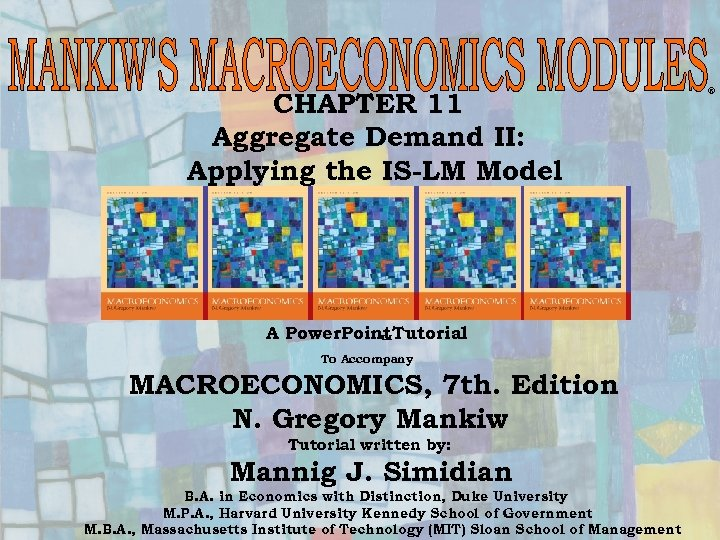CHAPTER 11 Aggregate Demand II: Applying the IS-LM Model A Power. Point Tutorial To Accompany MACROECONOMICS, 7 th. Edition N. Gregory Mankiw Tutorial written by: Mannig J. Simidian B. A. in Economics with Distinction, Duke University 1 M. P. A. , Harvard University Kennedy School of Government M. B. A. , Massachusetts Institute of Technology (MIT) Sloan School of Management Chapter Eleven ®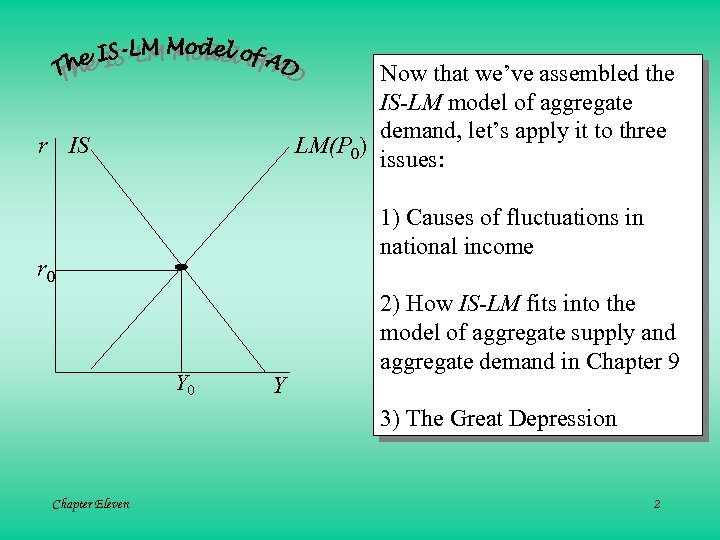Now that we’ve assembled the IS-LM model of aggregate demand, let’s apply it to three LM(P 0) issues: r IS 1) Causes of fluctuations in national income r 0 Y 2) How IS-LM fits into the model of aggregate supply and aggregate demand in Chapter 9 3) The Great Depression Chapter Eleven 2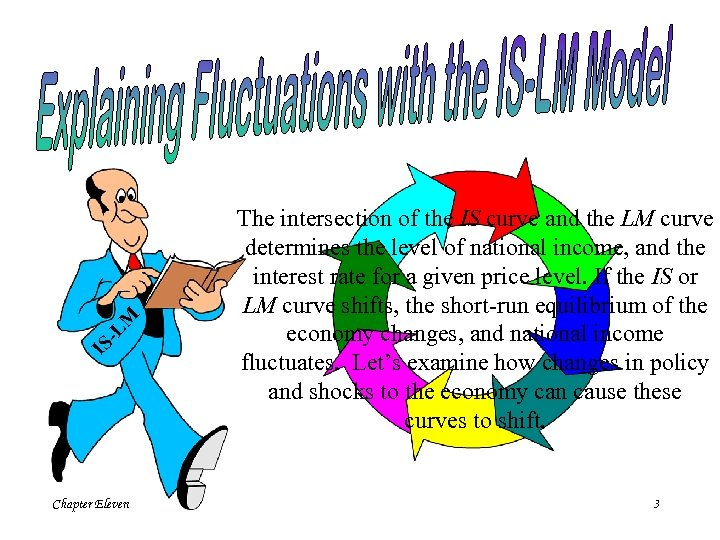-L M IS Chapter Eleven The intersection of the IS curve and the LM curve determines the level of national income, and the interest rate for a given price level. If the IS or LM curve shifts, the short-run equilibrium of the economy changes, and national income fluctuates. Let’s examine how changes in policy and shocks to the economy can cause these curves to shift. 3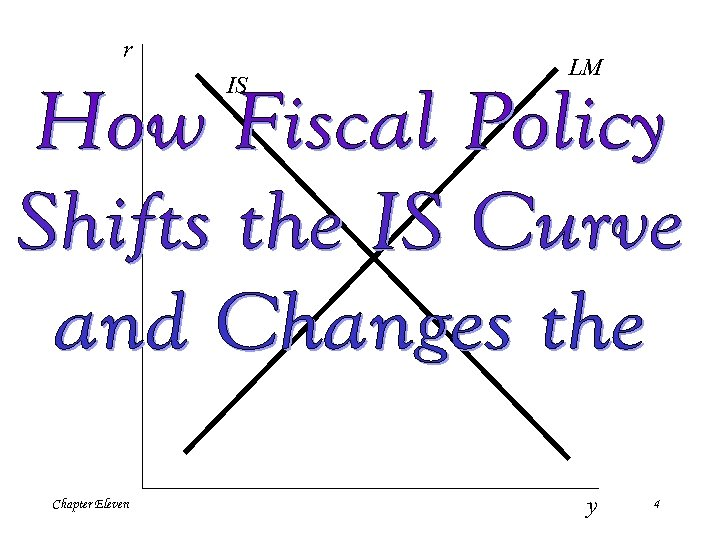r IS Chapter Eleven LM y 4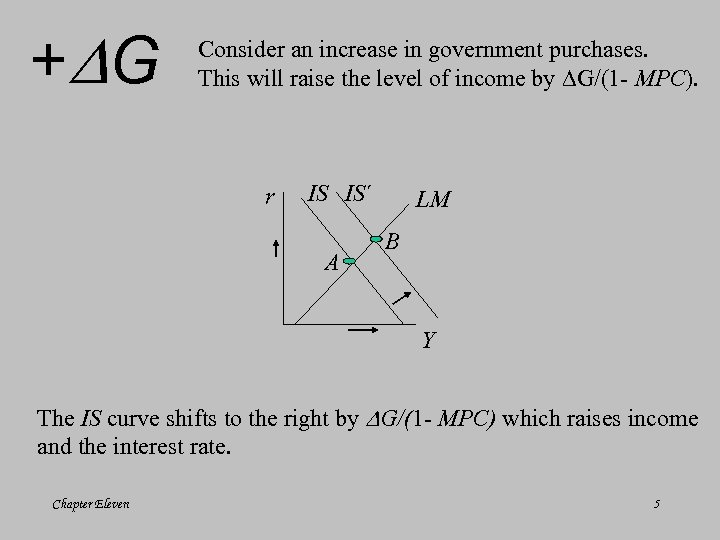+ G Consider an increase in government purchases. This will raise the level of income by G/(1 - MPC). r IS IS´ A LM B Y The IS curve shifts to the right by G/(1 - MPC) which raises income and the interest rate. Chapter Eleven 5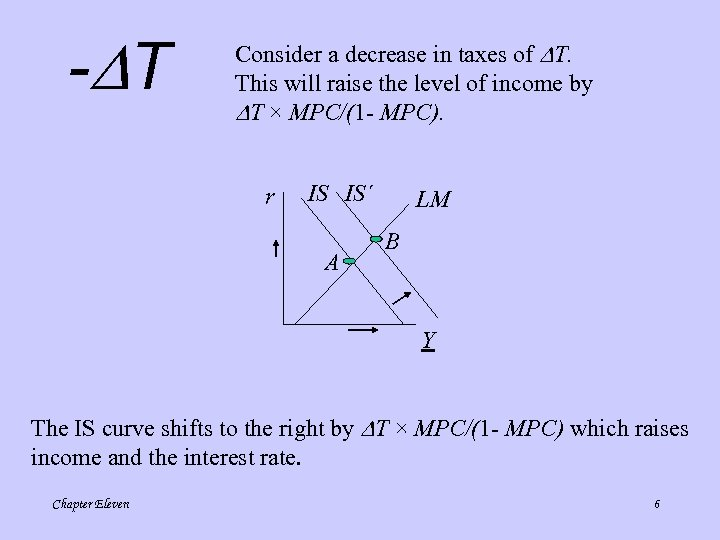- T Consider a decrease in taxes of T. This will raise the level of income by T × MPC/(1 - MPC). r IS IS´ A LM B Y The IS curve shifts to the right by T × MPC/(1 - MPC) which raises income and the interest rate. Chapter Eleven 6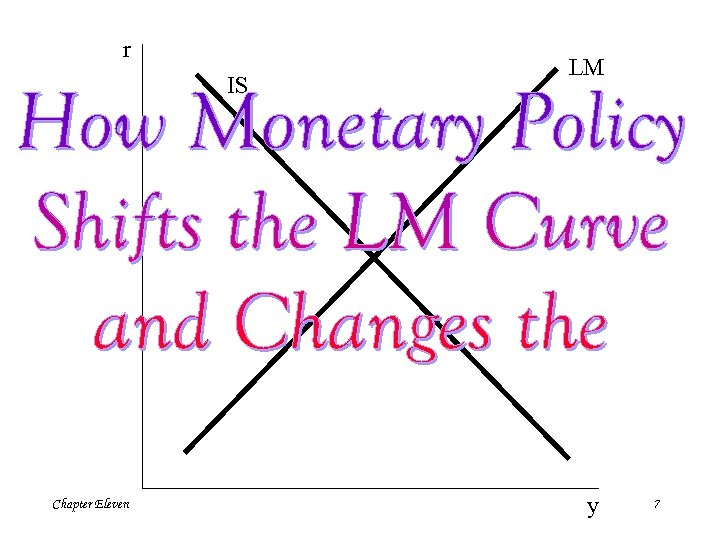r IS Chapter Eleven LM y 7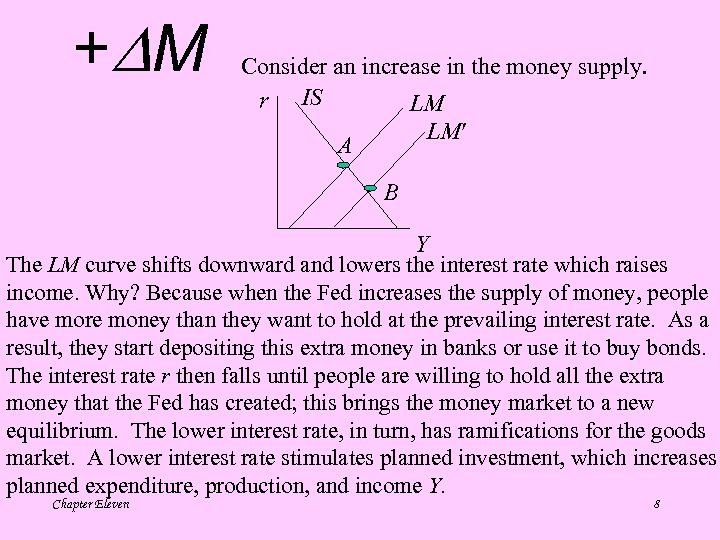+ M Consider an increase in the money supply. r IS LM LM A B Y The LM curve shifts downward and lowers the interest rate which raises income. Why? Because when the Fed increases the supply of money, people have more money than they want to hold at the prevailing interest rate. As a result, they start depositing this extra money in banks or use it to buy bonds. The interest rate r then falls until people are willing to hold all the extra money that the Fed has created; this brings the money market to a new equilibrium. The lower interest rate, in turn, has ramifications for the goods market. A lower interest rate stimulates planned investment, which increases planned expenditure, production, and income Y. Chapter Eleven 8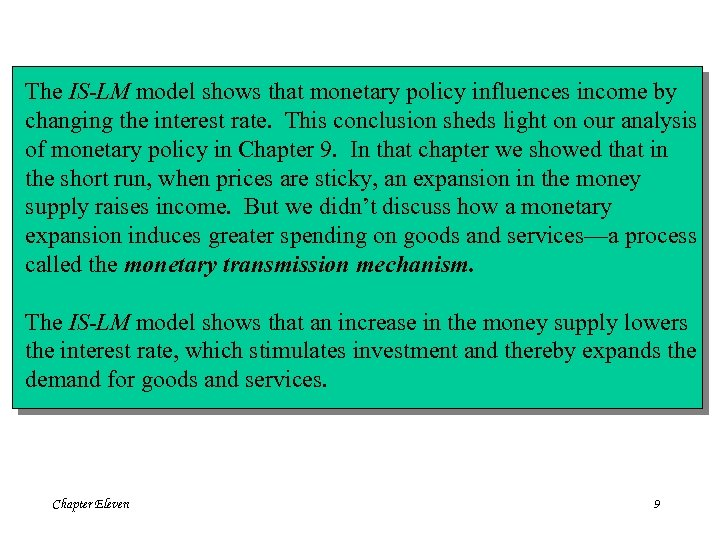The IS-LM model shows that monetary policy influences income by changing the interest rate. This conclusion sheds light on our analysis of monetary policy in Chapter 9. In that chapter we showed that in the short run, when prices are sticky, an expansion in the money supply raises income. But we didn’t discuss how a monetary expansion induces greater spending on goods and services—a process called the monetary transmission mechanism. The IS-LM model shows that an increase in the money supply lowers the interest rate, which stimulates investment and thereby expands the demand for goods and services. Chapter Eleven 9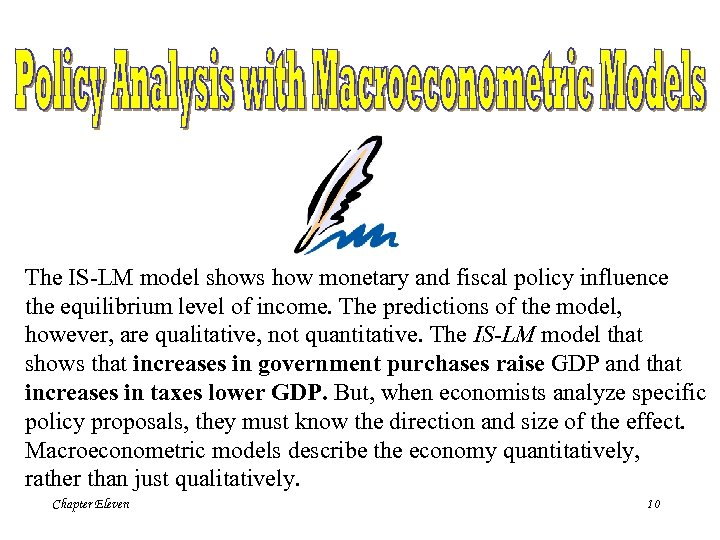The IS-LM model shows how monetary and fiscal policy influence the equilibrium level of income. The predictions of the model, however, are qualitative, not quantitative. The IS-LM model that shows that increases in government purchases raise GDP and that increases in taxes lower GDP. But, when economists analyze specific policy proposals, they must know the direction and size of the effect. Macroeconometric models describe the economy quantitatively, rather than just qualitatively. Chapter Eleven 10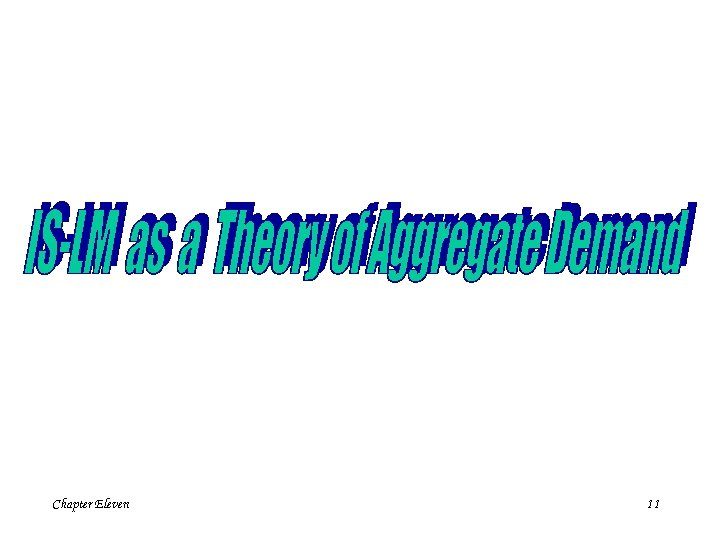Chapter Eleven 11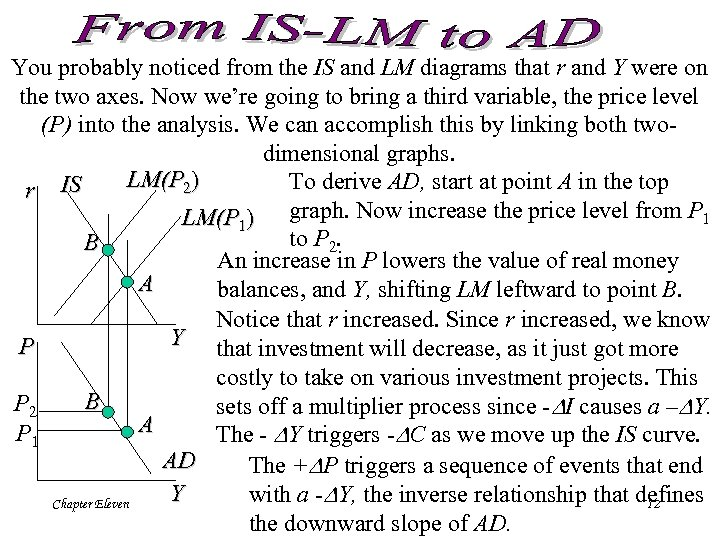You probably noticed from the IS and LM diagrams that r and Y were on the two axes. Now we’re going to bring a third variable, the price level (P) into the analysis. We can accomplish this by linking both twodimensional graphs. LM(P 2) To derive AD, start at point A in the top r IS LM(P 1) graph. Now increase the price level from P 1 to P 2. B An increase in P lowers the value of real money A balances, and Y, shifting LM leftward to point B. Notice that r increased. Since r increased, we know Y that investment will decrease, as it just got more P costly to take on various investment projects. This B P 2 sets off a multiplier process since - I causes a – Y. A P 1 The - Y triggers - C as we move up the IS curve. AD The + P triggers a sequence of events that end Y with a - Y, the inverse relationship that defines Chapter Eleven 12 the downward slope of AD.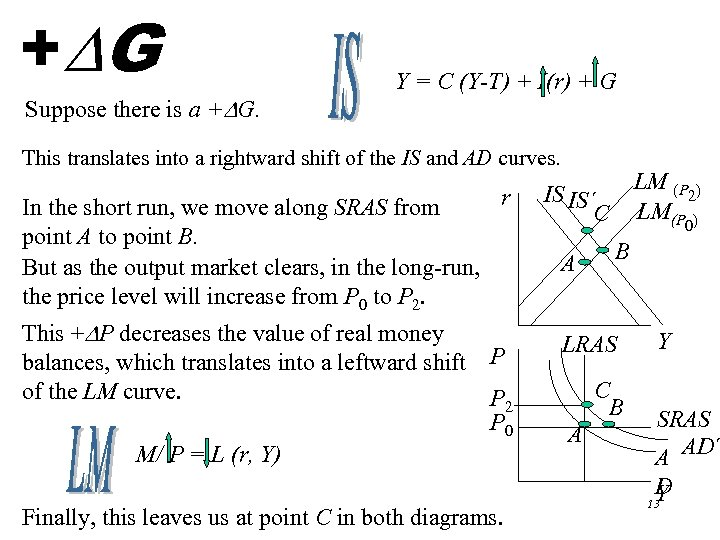+ G Suppose there is a + G. Y = C (Y-T) + I(r) + G This translates into a rightward shift of the IS and AD curves. r In the short run, we move along SRAS from point A to point B. But as the output market clears, in the long-run, the price level will increase from P 0 to P 2. This + P decreases the value of real money balances, which translates into a leftward shift P of the LM curve. P 2 P 0 M/ P = L (r, Y) Chapter Eleven Finally, this leaves us at point C in both diagrams. LM (P ) 2 LM(P ) IS IS´ C A 0 B LRAS C B A Y SRAS A AD´ D Y 13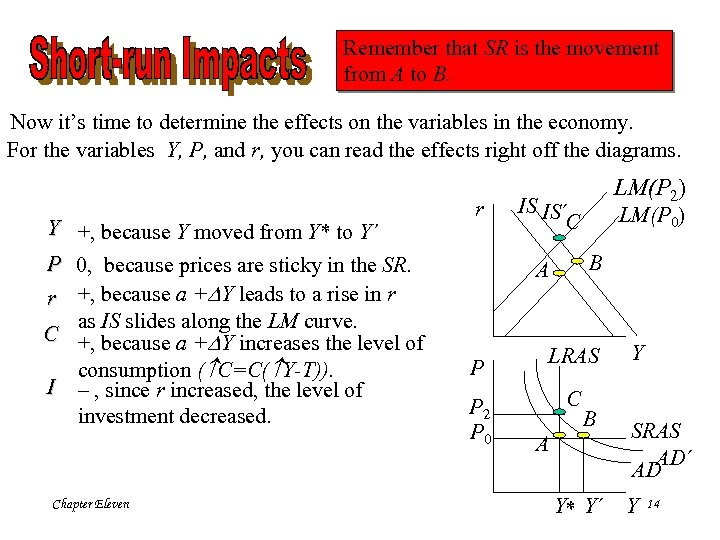Remember that SR is the movement from A to B. Now it’s time to determine the effects on the variables in the economy. For the variables Y, P, and r, you can read the effects right off the diagrams. Y +, because Y moved from Y* to Y´ P 0, because prices are sticky in the SR. r +, because a + Y leads to a rise in r as IS slides along the LM curve. C +, because a + Y increases the level of consumption ( C=C( Y-T)). I – , since r increased, the level of investment decreased. Chapter Eleven r IS IS´ C P 2 P 0 LM(P 0) B A P LM(P 2) LRAS C B A Y* Y´ Y SRAS AD´ AD Y 14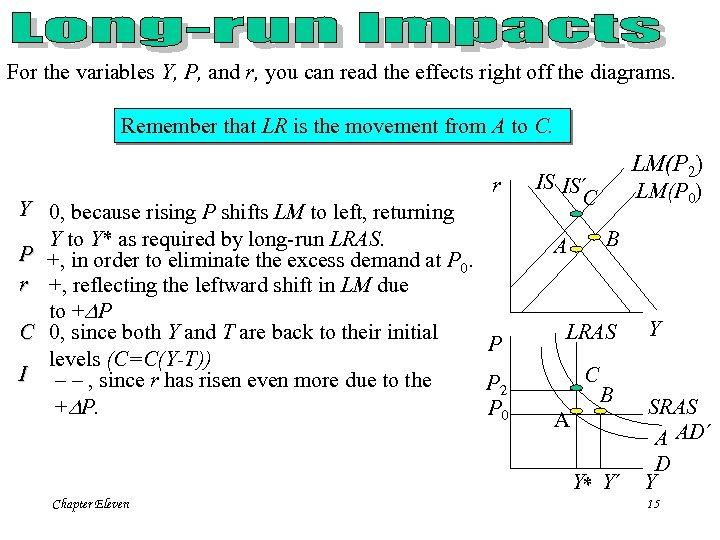For the variables Y, P, and r, you can read the effects right off the diagrams. Remember that LR is the movement from A to C. r Y 0, because rising P shifts LM to left, returning Y to Y* as required by long-run LRAS. P +, in order to eliminate the excess demand at P. 0 r +, reflecting the leftward shift in LM due to + P C 0, since both Y and T are back to their initial P levels (C=C(Y-T)) I – – , since r has risen even more due to the P 2 + P. P 0 Chapter Eleven LM(P 2) IS IS´ C LM(P 0) B A LRAS C B A Y* Y´ Y SRAS A AD´ D Y 15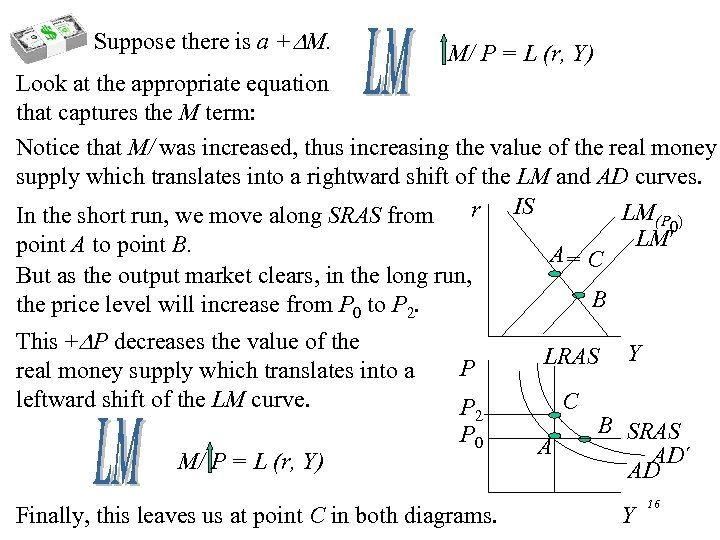Suppose there is a + M. M/ P = L (r, Y) Look at the appropriate equation that captures the M term: Notice that M/ was increased, thus increasing the value of the real money supply which translates into a rightward shift of the LM and AD curves. r IS LM(P ) In the short run, we move along SRAS from 0 LM point A to point B. A= C But as the output market clears, in the long run, B the price level will increase from P 0 to P 2. This + P decreases the value of the real money supply which translates into a leftward shift of the LM curve. M/ P = L (r, Y) Chapter Eleven P LRAS P 2 P 0 C Finally, this leaves us at point C in both diagrams. A Y B SRAS AD´ AD Y 16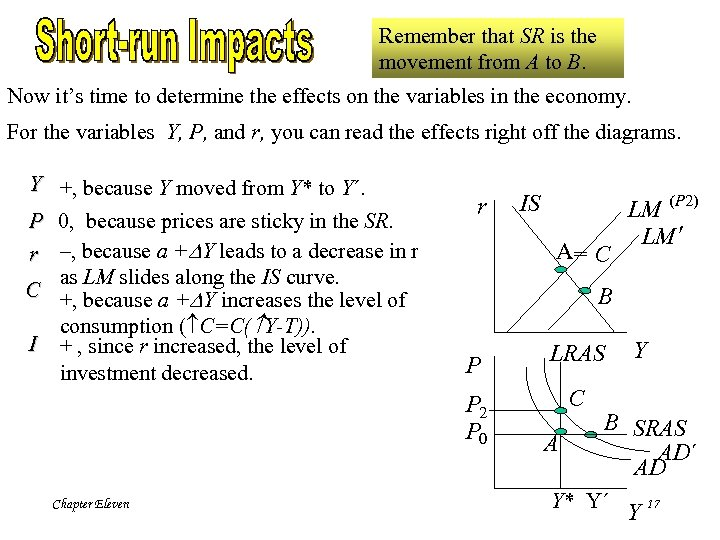Remember that SR is the movement from A to B. Now it’s time to determine the effects on the variables in the economy. For the variables Y, P, and r, you can read the effects right off the diagrams. Y +, because Y moved from Y* to Y´. P 0, because prices are sticky in the SR. r –, because a + Y leads to a decrease in r as LM slides along the IS curve. C +, because a + Y increases the level of consumption ( C=C( Y-T)). I + , since r increased, the level of investment decreased. r IS A= C B P LRAS P 2 P 0 Chapter Eleven (P 2) LM (P 0) LM C A Y B SRAS AD´ AD Y* Y´ Y 17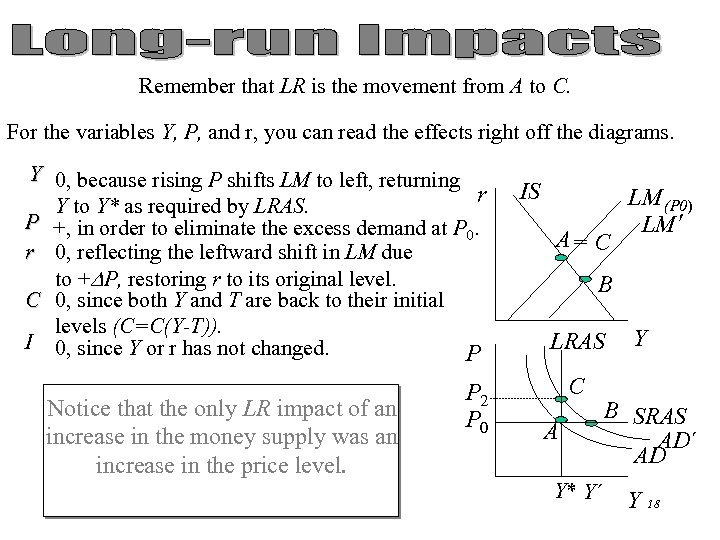Remember that LR is the movement from A to C. For the variables Y, P, and r, you can read the effects right off the diagrams. Y 0, because rising P shifts LM to left, returning r Y to Y* as required by LRAS. P +, in order to eliminate the excess demand at P. 0 r 0, reflecting the leftward shift in LM due to + P, restoring r to its original level. C 0, since both Y and T are back to their initial levels (C=C(Y-T)). I 0, since Y or r has not changed. P Notice that the only LR impact of an increase in the money supply was an increase in the price level. Chapter Eleven P 2 P 0 IS A= C LM (P 0) LM B LRAS C A Y* Y´ Y B SRAS AD´ AD Y 18Chapter Eleven 19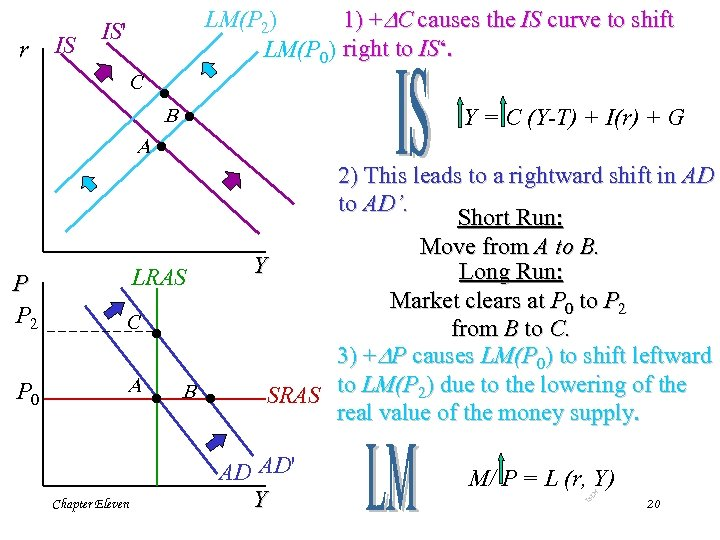C · B· Y = C (Y-T) + I(r) + G A· P P 2 P 0 LRAS C · A · B · Chapter Eleven 2) This leads to a rightward shift in AD to AD’. Short Run: Move from A to B. Y Long Run: Market clears at P 0 to P 2 from B to C. 3) + P causes LM(P 0) to shift leftward SRAS to LM(P 2) due to the lowering of the real value of the money supply. AD AD' Y M/ P = L (r, Y) -L M IS IS r LM(P 2) 1) + C causes the IS curve to shift LM(P 0) right to IS‘. IS' 20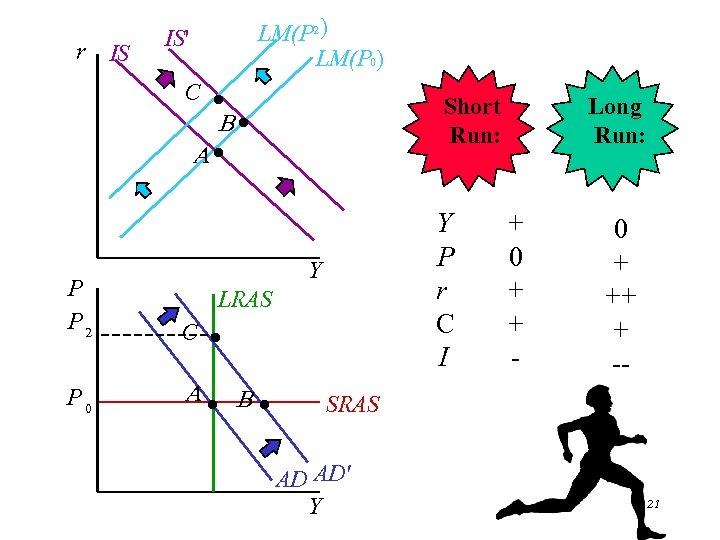r IS LM(P 2) LM(P 0) IS' C · Short Run: B· A· Y P r C I Y P P 2 C · P 0 A· B· Chapter Eleven LRAS Long Run: + 0 + + - 0 + ++ + -- SRAS AD AD' Y 21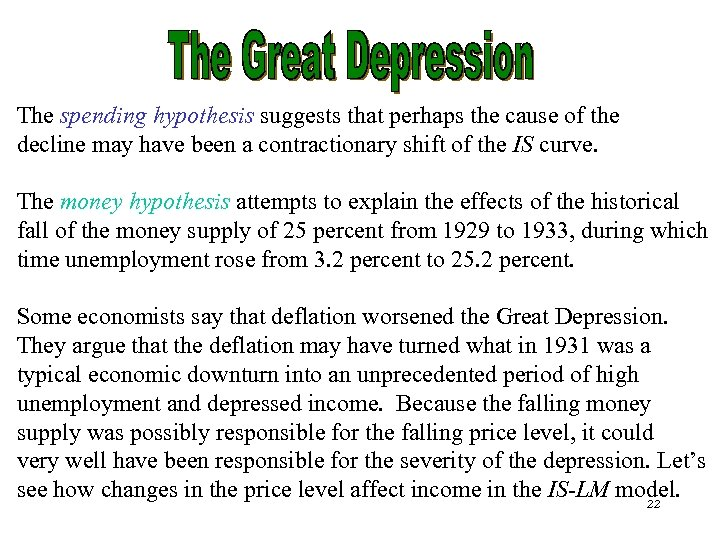The spending hypothesis suggests that perhaps the cause of the decline may have been a contractionary shift of the IS curve. The money hypothesis attempts to explain the effects of the historical fall of the money supply of 25 percent from 1929 to 1933, during which time unemployment rose from 3. 2 percent to 25. 2 percent. Some economists say that deflation worsened the Great Depression. They argue that the deflation may have turned what in 1931 was a typical economic downturn into an unprecedented period of high unemployment and depressed income. Because the falling money supply was possibly responsible for the falling price level, it could very well have been responsible for the severity of the depression. Let’s see Chapter Eleven how changes in the price level affect income in the IS-LM model. 22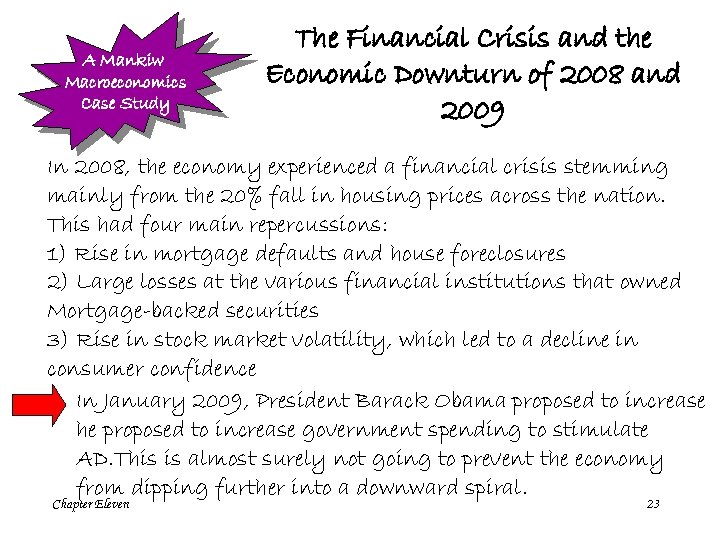A Mankiw Macroeconomics Case Study The Financial Crisis and the Economic Downturn of 2008 and 2009 In 2008, the economy experienced a financial crisis stemming mainly from the 20% fall in housing prices across the nation. This had four main repercussions: 1) Rise in mortgage defaults and house foreclosures 2) Large losses at the various financial institutions that owned Mortgage-backed securities 3) Rise in stock market volatility, which led to a decline in consumer confidence In January 2009, President Barack Obama proposed to increase he proposed to increase government spending to stimulate AD. This is almost surely not going to prevent the economy from dipping further into a downward spiral. Chapter Eleven 23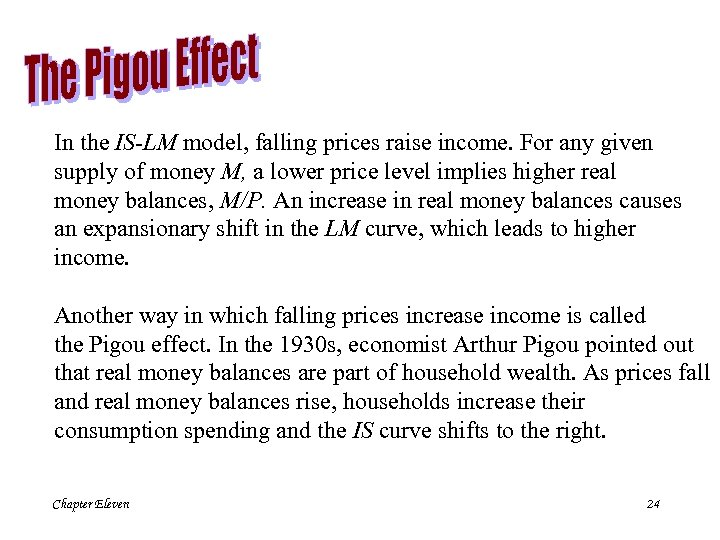In the IS-LM model, falling prices raise income. For any given supply of money M, a lower price level implies higher real money balances, M/P. An increase in real money balances causes an expansionary shift in the LM curve, which leads to higher income. Another way in which falling prices increase income is called the Pigou effect. In the 1930 s, economist Arthur Pigou pointed out that real money balances are part of household wealth. As prices fall and real money balances rise, households increase their consumption spending and the IS curve shifts to the right. Chapter Eleven 24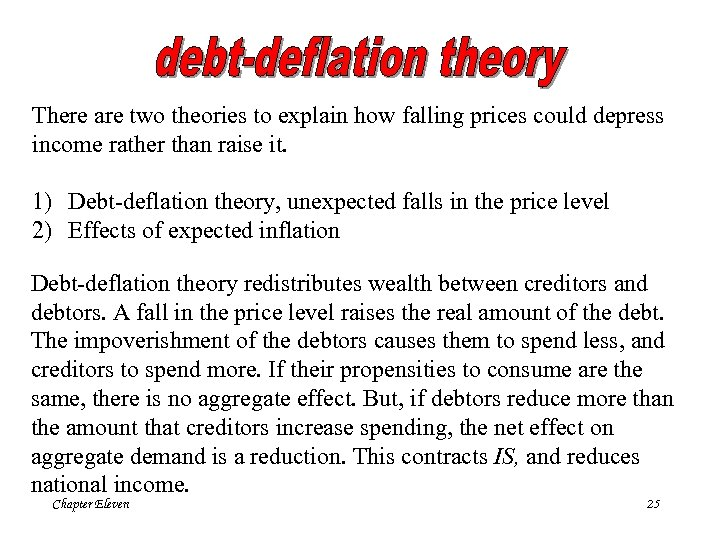There are two theories to explain how falling prices could depress income rather than raise it. 1) Debt-deflation theory, unexpected falls in the price level 2) Effects of expected inflation Debt-deflation theory redistributes wealth between creditors and debtors. A fall in the price level raises the real amount of the debt. The impoverishment of the debtors causes them to spend less, and creditors to spend more. If their propensities to consume are the same, there is no aggregate effect. But, if debtors reduce more than the amount that creditors increase spending, the net effect on aggregate demand is a reduction. This contracts IS, and reduces national income. Chapter Eleven 25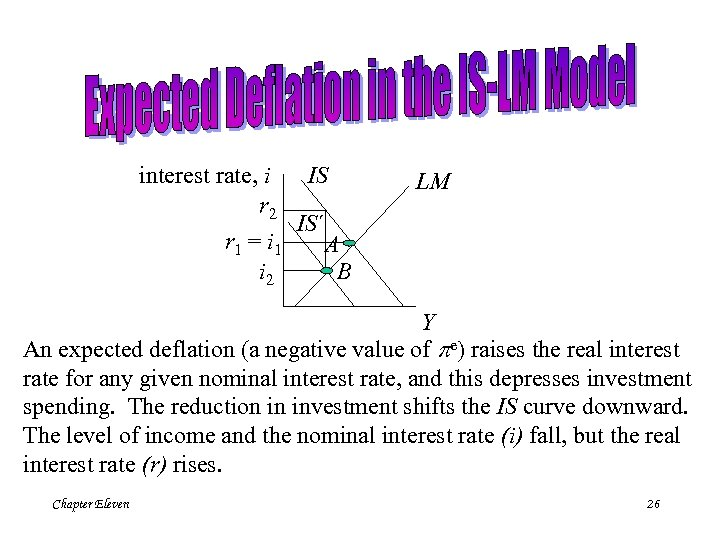IS interest rate, i r 2 IS´ r 1 = i 1 A i 2 B LM Y An expected deflation (a negative value of pe) raises the real interest rate for any given nominal interest rate, and this depresses investment spending. The reduction in investment shifts the IS curve downward. The level of income and the nominal interest rate (i) fall, but the real interest rate (r) rises. Chapter Eleven 26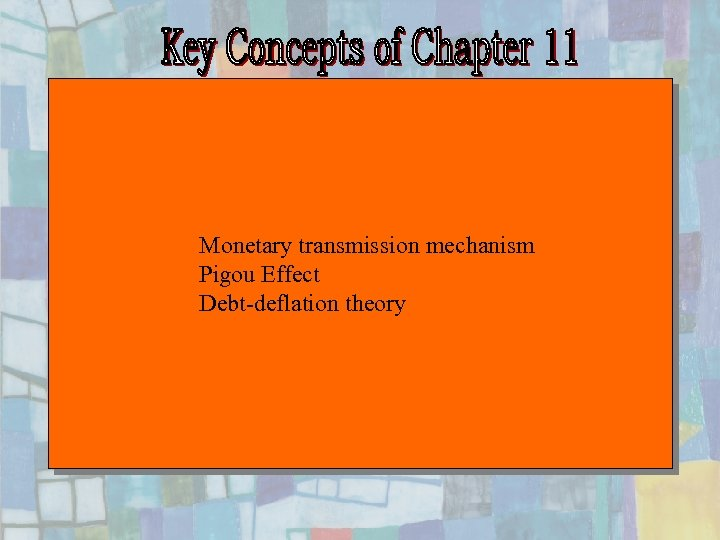Monetary transmission mechanism Pigou Effect Debt-deflation theory Chapter Eleven 27